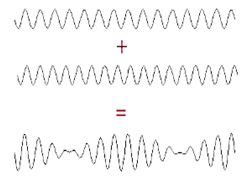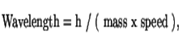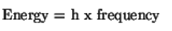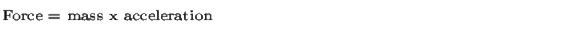Wave-Particle Duality:

Introduction:The Bohr model of the atom involved two puzzling features - the electron was treated as a wave, and light was treated as a particle (a photon).

Explanation:

The connection to these new pictures of electrons and light from our more familiar view of an electron as a particle and light as a wave is provided by the relation.Which links the mass and speed of an electron to the wavelength of the associated wave, and by the relationWhich links the frequency of a light wave to the energy of the associated photon.

These relations are not derivable from other relations; they are hypothesized, and are ``true'' only so long as they satisfy experimental verification. Even so, they are unlike most mathematical statements, however, in the following sense. A statement like Newton's 2nd law of motion,Refers on both sides of the equal sign to the same object: one says the force on some object is equal to the mass of the object times the acceleration of the object. Implicit in this is the fact that both sides of the equation are referring to the same object, and so we have in mind one common picture of the object. However, the preceding quantum relations are referring to differentpictures of an object: on one side of the equation we view the object as a particle, and use words to describe the particle's mass, speed, and energy, and on the other side of the equation we view the object as a wave, and use words like wavelength and frequency to describe that wave.